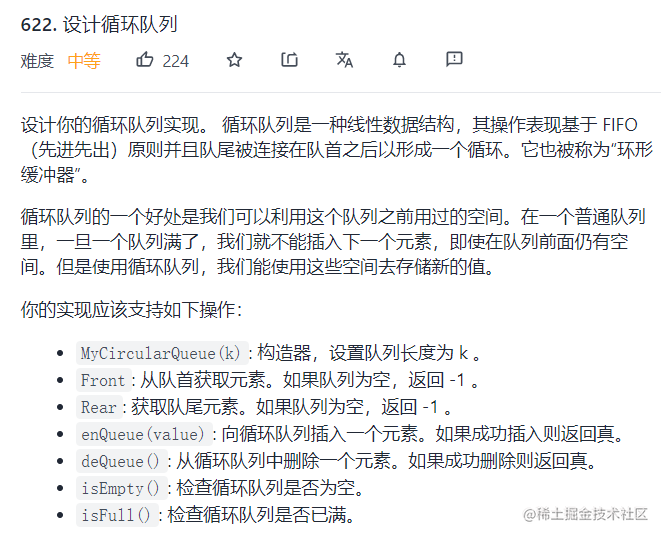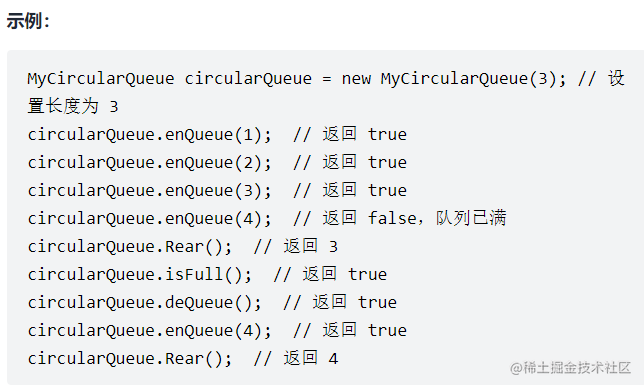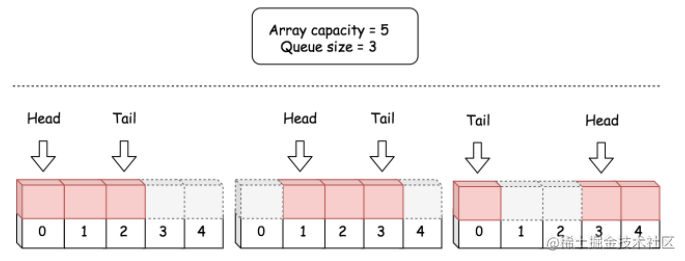# 题目介绍# 方法一：数组• `queue`：一个固定大小的数组，用于保存循环队列的元素。

• `headIndex`：一个整数，保存队首 `head` 的索引。

• `count`：循环队列当前的长度，即循环队列中的元素数量。使用 `hadIndex``count` 可以计算出队尾元素的索引，因此不需要队尾属性。

• `capacity`：循环队列的容量，即队列中最多可以容纳的元素数量。该属性不是必需的，因为队列容量可以通过数组属性得到，但是由于该属性经常使用，所以我们选择保留它。这样可以不用在 Python 中每次调用 len(queue) 中获取容量。但是在 Java 中通过 queue.length 获取容量更加高效。为了保持一致性，在两种方案中都保留该属性。

``````class MyCircularQueue {
private int[] queue;//存储队列元素
private int count;//当前队列节点个数
private int capacity;//当前队列的空间大小

/** Initialize your data structure here. Set the size of the queue to be k. */
public MyCircularQueue(int k) {
this.capacity = k;
this.queue = new int[k];
this.count = 0;
}

/**
* 进队列
*/
public boolean enQueue(int value) {
//队列已满
if (this.count == this.capacity)
return false;
//计算出尾索引的下一个索引
this.queue[(this.headIndex + this.count) % this.capacity] = value;
this.count += 1;
return true;
}

/**
* 出队列
*/
public boolean deQueue() {
//队列元素为空
if (this.count == 0)
return false;
//调整头索引
//队列元素个数减一
this.count -= 1;
return true;
}

/**
* 获取头元素，即头索引对应的元素
*/
public int Front() {
if (this.count == 0)
return -1;
}

/**
* 获取尾元素，即尾索引对应的元素
*/
public int Rear() {
if (this.count == 0)
return -1;
int tailIndex = (this.headIndex + this.count - 1) % this.capacity;
return this.queue[tailIndex];
}

/**
* 判断队列是否为空
*/
public boolean isEmpty() {
return (this.count == 0);
}

/**
* 判断队列是否已满
*/
public boolean isFull() {
return (this.count == this.capacity);
}
}

``````public class MyCircularQueue {
private int[] data; // 循环队列的长度固定，直接用数组即可
private int tail;
private int size;

public MyCircularQueue(int k) { // 构造方法
data = new int[k];
tail = -1;
size = k;
}

/** 将元素插入循环队列。如果操作成功，则返回true */
public boolean enQueue(int value) {
// 如果队列已满，则无法成功添加新元素，返回false
if (isFull()) {
return false;
}
if (isEmpty()) {
}
tail = (tail + 1) % size; // 计算下一个tail的值
data[tail] = value;
return true;
}

/** 从循环队列中删除一个元素。如果操作成功，则返回true */
public boolean deQueue() {
// 如果队列为空，则无法成功删除元素，返回false
if (isEmpty()) {
return false;
}
if (head == tail) { // 只有一个元素的情况
tail = -1;
} else {
}
return true;
}

/** 从队首获取元素。如果队列为空，返回 -1 */
public int Front() {
if (isEmpty()) {
return -1;
}
}

/** 获取队尾元素。如果队列为空，返回 -1 */
public int Rear() {
if (isEmpty()) {
return -1;
}
return data[tail];
}

/** 检查循环队列是否为空 */
public boolean isEmpty() {
}

/** 检查循环队列是否为满 */
public boolean isFull() {
return (tail + 1) % size == head;
}
}

• 时间复杂度： O(1)。该数据结构中，所有方法都具有恒定的时间复杂度。

• 空间复杂度： O(N)，其中 N 是队列的预分配容量。循环队列的整个生命周期中，都持有该预分配的空间。